# Blog

## PieceWise Functions NYS Regents: Algebra

Greetings math friends, students, and teachers I come in peace to review this piecewise functions NYS Regents question.  Are they pieces of functions? Yes. Are they wise?  Ah, yeah sure, why not? Let’s check out this piecewise functions NYS Regents question below and happy calculating! 🙂

1 value satisfies the equation because there is only one point on the graph where f(x) and g(x) meet.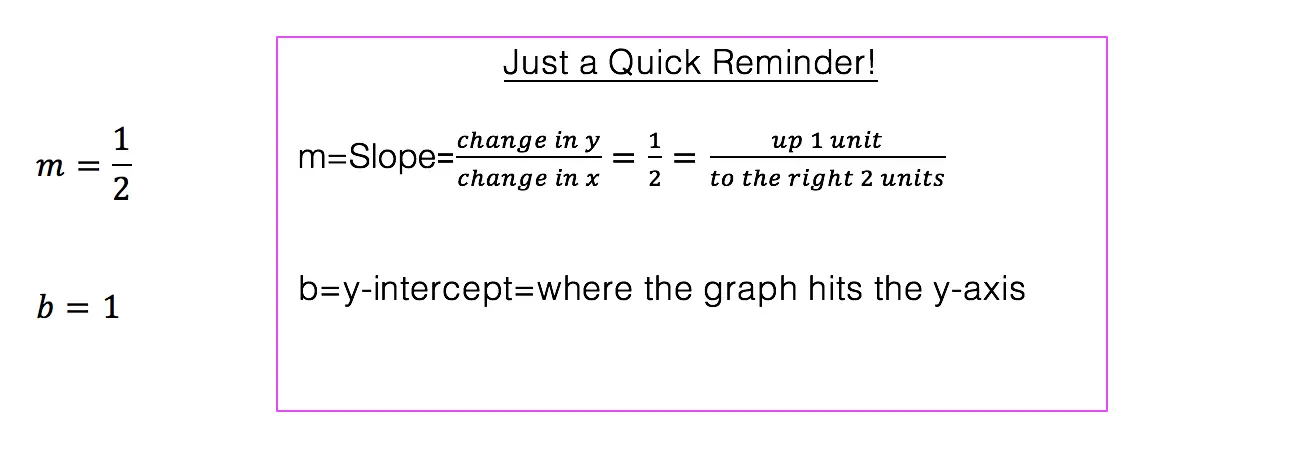*Note: For an in-depth review on how to graph an equation of a line step by step, check out this post here.

Does the above madness make sense to you? Great!

Need more of an explanation? Keep going! There is a way to understand the above mess.

Looking at this piece-wise function: We want to graph the function 2x+1 but only when the x-values are less than or equal to negative 1.

We also want to graph 2-x^2 but only when x is greater than the negative one.

One way to organize graphing each piece of a piecewise function is by making a chart.

1. Lets start by making a chart for the first part of our function 2x+1:

Is it all coming back to you now?  Need more practice on piece-wise functions?Check out this link here and happy calculating! 🙂

Also, if you’re looking to nourish your mind or you know, procrastinate a bit check out and follow MathSux on these websites!

## Top 5 Favorite Math Websites

As we all love technology (I’m assuming since it has brought you here), I figured I should share my top favorite math sites.  These are great resources for learning things you don’t understand, practicing for an upcoming test or if you’re like me, it’s great for re-learning/teaching purposes.  Yes, even I forget how to do things and these websites are a great help.  I know the anticipation is getting to you so I’ll just get to the good stuff now. Here are my top 5 favorite math websites:

1. Khan Academy : This is a non profit over in Silicon Valley.  They have a great range of subjects (k-college level)  and explain everything via easy to follow videos.
2. Regent’s Prep: The title is pretty self explanatory, but this helps with reviewing for the New York State Regent’s.  This site has a lot of great examples and answers.
3.  MathSux:  So fabulous, no explanation is required. 😀
4.  Knewton:  Pretty cool things are happening at this web address.  You can make an account, answer some questions and the questions will adjust according to your answers figuring out what you need help in.
5. Kahoot: Fast and easy quizzes that are good for an entire classroom or for studying alone.  Either way there are lots of bright and happy colors that make you forget you’re studying anything in the first place.

So those are the goods, the contraband, the info you can’t find anywhere else (all lies).  Hope you find these websites useful. Let me know if you end up using any of them.  🙂

## Pre-Calc: The Factor Theorem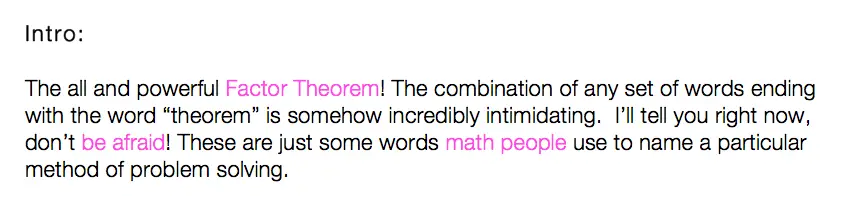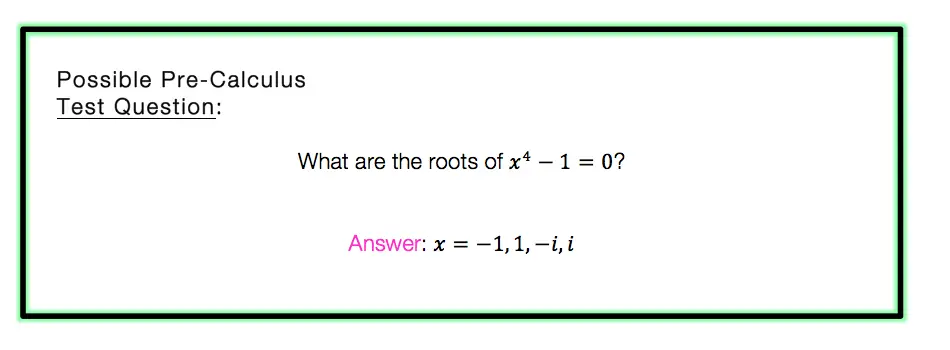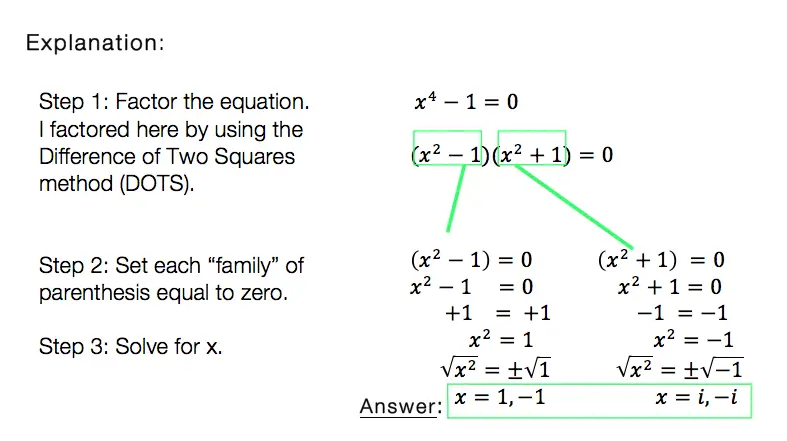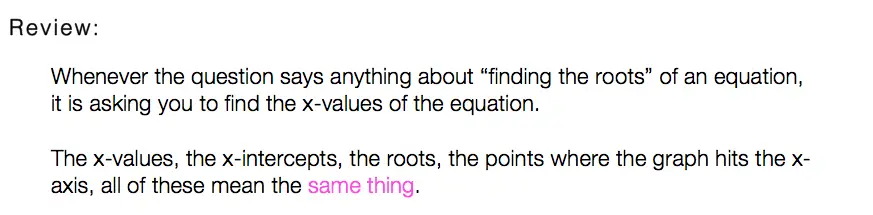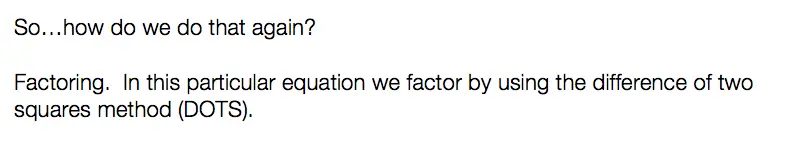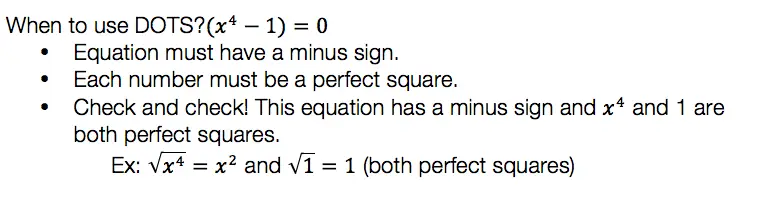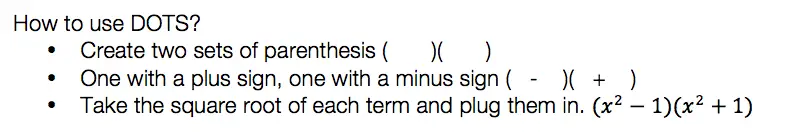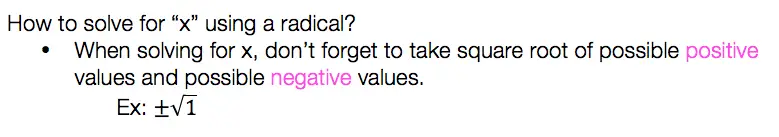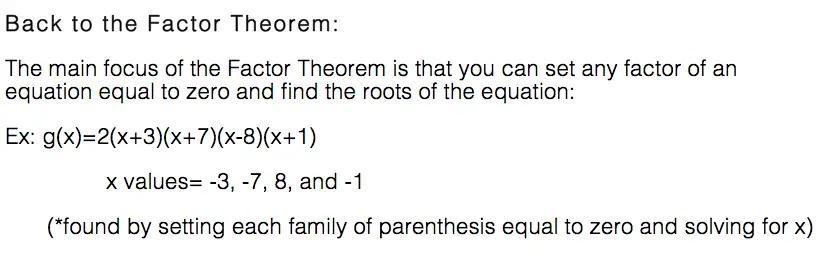So what do we think of the factor theorem?  Not as intimidating as you thought, you probably have been using it for years without realizing it.

For more math fun check me out on Twitter and Facebook.  Until next time, keep it real and happy mathing! 🙂

For related posts on factoring methods that apply the factor theorem, check the following lessons out below:

Why must we complete the square?

4 Ways to Factor a Trinomial

Factor By Grouping

## Factor by Grouping: Algebra 2/Trig.

Hey math friends! In this post, we are going to go over Factor by Grouping, one of the many methods for factoring a quadratic equation.  There are so many methods to factor quadratic equations, but this is a great choice, for when a is greater than 1. Also, if you need to review different types of factoring methods, just check out this link here.  Stay curious and happy calculating! 🙂 Before we go any further, let’s just take a quick look at what a quadratic equation is: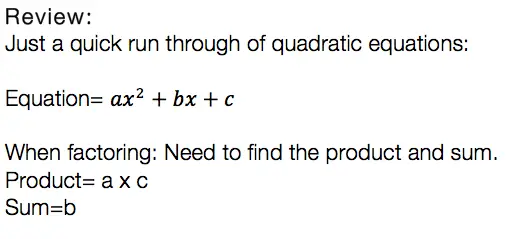Usually, we can just find the products, the sum, re-write the equation, solve for x, and be on our merry way.  But if you notice, there is something special about the question below. The coefficient “a” is greater than 1/. This is where factor by grouping comes in handy!Now that we know why and when we need to factor by grouping lets take a look at our Example: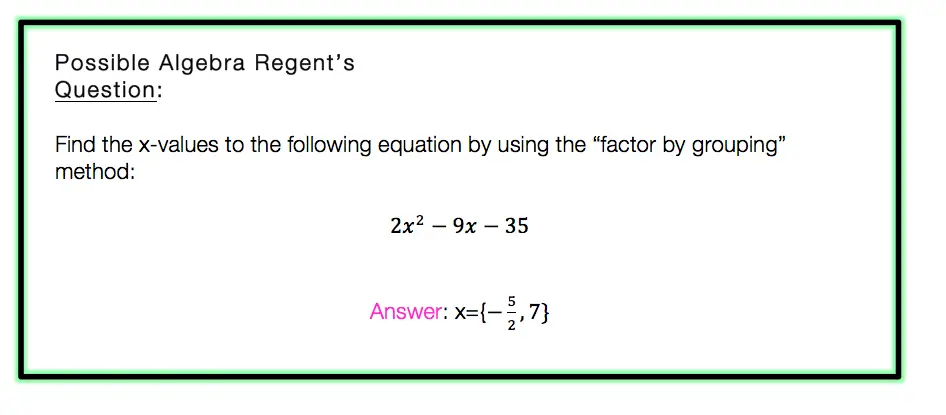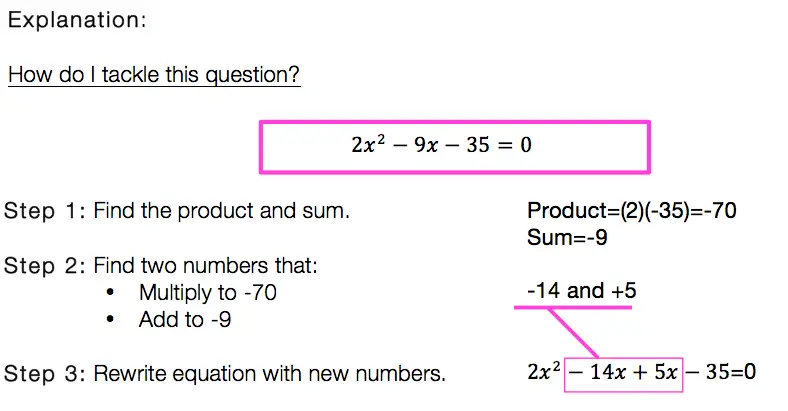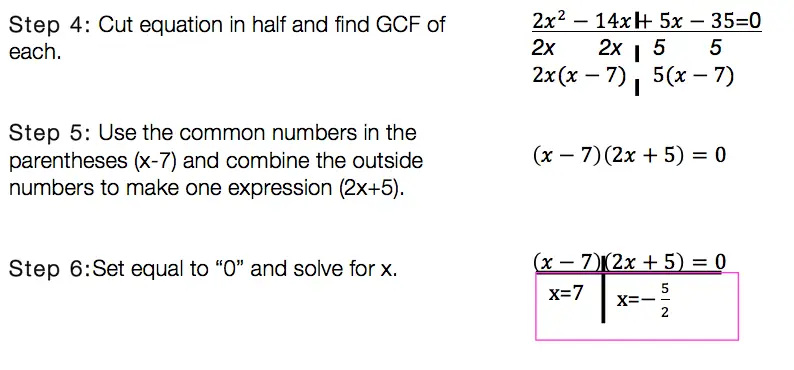Factor By Grouping: Hard to solve? No.  Hard to remember? It can be, just remember to practice, practice practice! Also, if you are in need of a review of other methods of factoring quadratic equations, click this link here.

Want more Mathsux?  Don’t forget to check out our Youtube channel and more below! And if you have any questions, please don’t hesitate to comment. Happy Calculating! 🙂

Looking for more on Quadratic Equations and Functions? Check out the following Related posts!

Factoring Review

What is the Discriminant?

Completing the Square

4 Ways to Factor a Trinomial

Is it a Function?

Imaginary and Complex Numbers

Quadratic Equations with 2 Imaginary Solutions

Focus and Directrix of a Parabola

## Equation of A Circle: Geometry

Equation of A Circle

Ahoy math friends and welcome to Math Sux! In this post, we are going to learn about the equation of a circle, mainly how to write it when given a circle on a coordinate plane. We’ll see how to find the radius and center from the graphed circle and then see how to transfer that into our equation of a circle. And we’re going to do all of this step by step with the following Regents question. Stay curious and happy calculating! 🙂

## How do I Answer this Question?

Let’s mark up this coordinate plane and take as much information away from it as possible. When looking at this coordinate plane, we can find the value of the circle’s center and the circle’s radius.

Now that found the center and radius, we can fit each into our equation:

Center=(-3,-4)

The only equation that matches our center and radius, is choice (2).

If the above answer makes sense to you, that’s great! If you need a little further explanation, keep going!

## Equation of a Circle:

Equation of a Circle: Let’s take a look at our answer and break down what each part means:

So what do you think of circles now?  Not to shabby, ehh?  🙂

Looking for more on circles?  Check out this post on how to find the Area of a Sector here!

## Recursive Formula: Algebra

Howdy math peeps! In this post, we are going to go over the recursive formula step by step by reviewing an old Regent’s question. These things may look weird, confusing, and like a “what am I doing?” moment, but trust me they are not so bad! We are going to take a look at the Regents question below and then find the correct recursive formula that follows the given sequence by going through each answer choice. Before we begin to answer our questions though, we will first define and break down what a recursive sequence is. Also, be sure to check out the video at the end of this post for more examples and further explanation. Happy calculating!

Before we dive into the solution to this question, let’s first look at a recursive formula example and define what they are in the first place!

## What is the Recursive Formula?

A Recursive Formula is a formula that forms a sequence based on the previous term value. All this means is that it uses a formula to form a sequence-based pattern. Recursive formulas can take the shape of different types of sequences, including arithmetic sequences (sequence based on adding/subtracting numbers) or geometric sequences (sequence based on multiplying/dividing numbers). Check out the example below:

a1=2 , an+1=an+4

## How do You Solve a Recursive Equation?

When solving a recursive equation, we are always given the first term and a formula. We start by using the first given term of our sequence, usually represented as a1, and plug it into the given formula to find the value of the second term of the sequence. Then we take the value of the previous term (the second term in our sequence) and plug it into our formula again, to find the third value of our sequence…. and the pattern continues! The solutions we get from each step up forms a sequence. If this sounds confusing, don’t worry because we are going to look at an example! Take a look at the recursive formula below:

a1=2 , an+1=an+4

Now let’s take another look at our recursive formula, this time breaking down what each part means:

a1 always represents the first given term, which in this case is a1=2. Next, we plug in 2 for an into our formula an+4 to get (2)+4=6. This gives us our second term in the sequence, which is 6. Next, we plug in 6 into the formula to get (6)+4=10, which is the value of the third term in the sequence. And we continue the pattern, always taking the value of the preceding term! In this case, I just found the first few terms below, but the recursive formula can continue infinitely! Again , if this sounds confusing, please take look at the pattern below:

Terms of the Sequence: 2, 6, 10, 14, 18….

Notice all the terms of the sequence are circled in pink, forming a sequence of, 2, 6, 10, 14, 18!? This is what the recursive formula produces!

## Now back to our Original Question:

Q: What recursively-defined function represents the sequence 3, 7, 15, 31,

(1) f(1) = 3, f(n + 1) = 28 (n) +3

(2) f(1) = 3, f (m + 1) = 28(0) – 1

(3) f(1) = 3, f(n + 1) = 28 (n) +1

(4) f(1) = 3, f (n + 1) = 38 (n) -2

How do I answer this question?

At first glance, all of these answer choices may look exactly the same as there are many recursive formulas to choose from. The first thing we need to do is to identify how each answer choice is different. Notice below, the section highlighted in green? This is what we will focus on for finding the correct recursive formula!

The question we are working with actually gives us a sequence and we need to find the recursive formula that works with it! Our goal with this question is to work backward to test out each recursive formula given to us until we get the correct sequence. To begin, let’s first identify each term in our given sequence.

As we go through each answer choice, we are looking for the recursive formula that gives us the above sequence 3, 7, 15, 31. Let’s start with choice (1) which happens to be a type of geometric sequence.

Right away we can see the sequence forming for choice (1) is 3, 11, … where the first term, 3, matches our original sequence, but the second term we get which is, 11, does not. This means we will need to move on and find the sequence of the next option, choice (2), which happens to be another type of geometric sequence. Let’s take a look:

For choice (2), we can see that the sequence we get is 3, 7, 127 which matches our given sequence for the first two terms 3, 7, but does not match the third term 127, when we need a 15 here (the original sequence is 3,7,15,31). Thus, we must move onward to the next recursive formula by testing out choice (3), which this time is an arithmetic sequence! Maybe we will have better luck!

This last option, choice (3) provided us with the same sequence we were originally provided within the original question, 3, 7, 15, 31. We have found our answer and now we can celebrate!

## Recursive Formula Examples

Still have questions about recursive formulas? Check out more on recursive formula examples here and in the video above! And if you’re looking to learn all there is to know about sequences, check out this post here! Looking to move ahead? Check out the infinite geometric series lesson here! Also, please don’t hesitate to comment with any questions. Happy calculating! 🙂

## Solving Log Equations: Algebra 2/Trig.

Ahoy math friends! In this post, we are going to focus on solving log equations by solving this Regents questions step by step. We’ll answer this question right away! But if you need more of a review, keep reading and you will find what logarithms are, the different kinds of logarithms rules, and some simpler examples. Ready for our first example?! Check it out below:

## How do I Answer this Question?

Step 1: Let’s re-write the equation to get rid of the “log.”

Step 2: Solve for x in our new equation (5x-1)(1/3)=4

If the above answer makes sense to you, great! If not, that’s ok too, keep reading for a review on solving log equations.

## What are Logarithms?

Logarithms are inverses of exponential equations. Take a look below for a clearer picture.

Basic Formula:

Logarithms can be re-written to get rid of the word “log.” This makes them easier to solve and understand.

Log Rules:

There are a few rules you have to memorize get used to with practice. These rules are used when solving for x in different kinds of algebraic log problems:

Still got questions?  No problem! Check out the videos below and the post here for more on logarithms! Also don’t forget to subscribe below to get the latest FREE math videos, lessons, and practice questions from MathSux. Thanks for stopping by and happy calculating! 🙂

Looking for another math challenge? Check out this post on solving 3 simultaneous equations at once!